## I am Abhiroop!

### MASTERS THESIS

Superword Level Parallelism in the Glasgow Haskell Compiler

### Single Instruction Multiple Data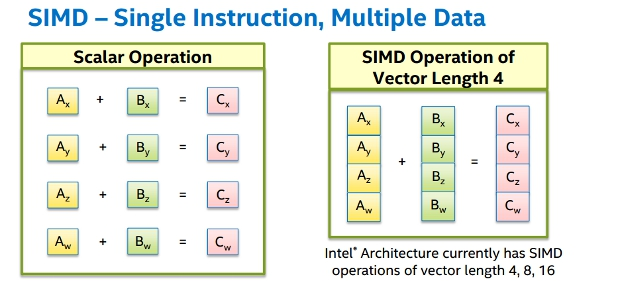### Motivation

• Power efficient
• Lesser FDE cycles for a given data size
• ML algorithms are data parallel in nature
• Numerical computing algorithms are data parallel

### OUTCOMES

• Support for SSE and AVX instructions in the NCG of GHC (Float and Double)
• Some core changes in `Data.Int`
• Flat Data Parallelism over SIMD registers in GHC (https://github.com/Abhiroop/lift-vector)

### FAILURES

• Automatic Vectorization

`ghc -ftree-vectorize Program.hs`

State of the art in LLVM : Porpodas, Vasileios, and Timothy M. Jones. "Throttling automatic vectorization: When less is more."

### POSSIBLE DIRECTIONS

• Experiment with SSA representation inside GHC
• Explore the SPMD on SIMD approach

Today's Talk:

## CONS LIST

• cdr (tail)
• cons

`cons (car xs) (cdr xs) = xs`

Cons List are sequential by construction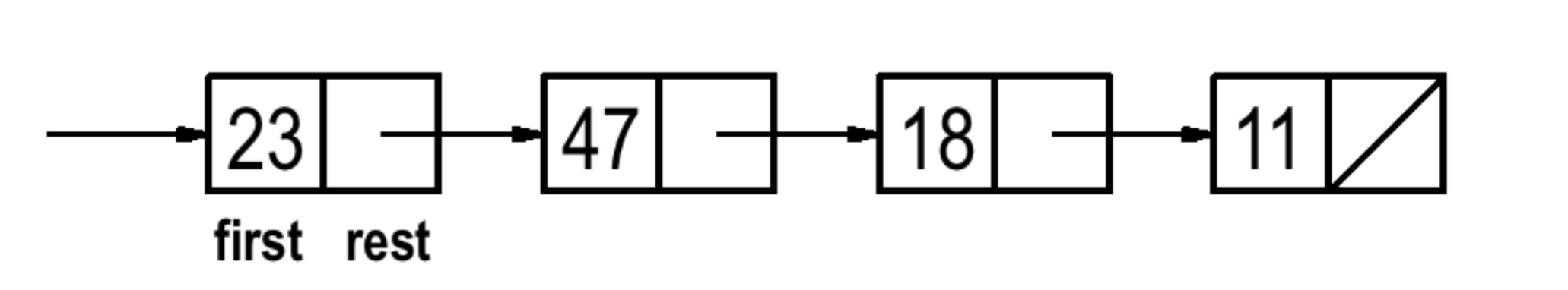`length` operation:

``````length :: [a] -> Int
length [] = 0
length (x:xs) = 1 + length xs``````

This is a parallelizable operation

``````length :: [a] -> Int
length []  = 0
length [a] = 1
length (a ++ b) = length a + length b``````

### Guy Steele - ICFP 2009

"foldl and foldr considered slightly harmful"

We must lose the “accumulator” paradigm and emphasize “divide-and-conquer”.

### CONC LISTS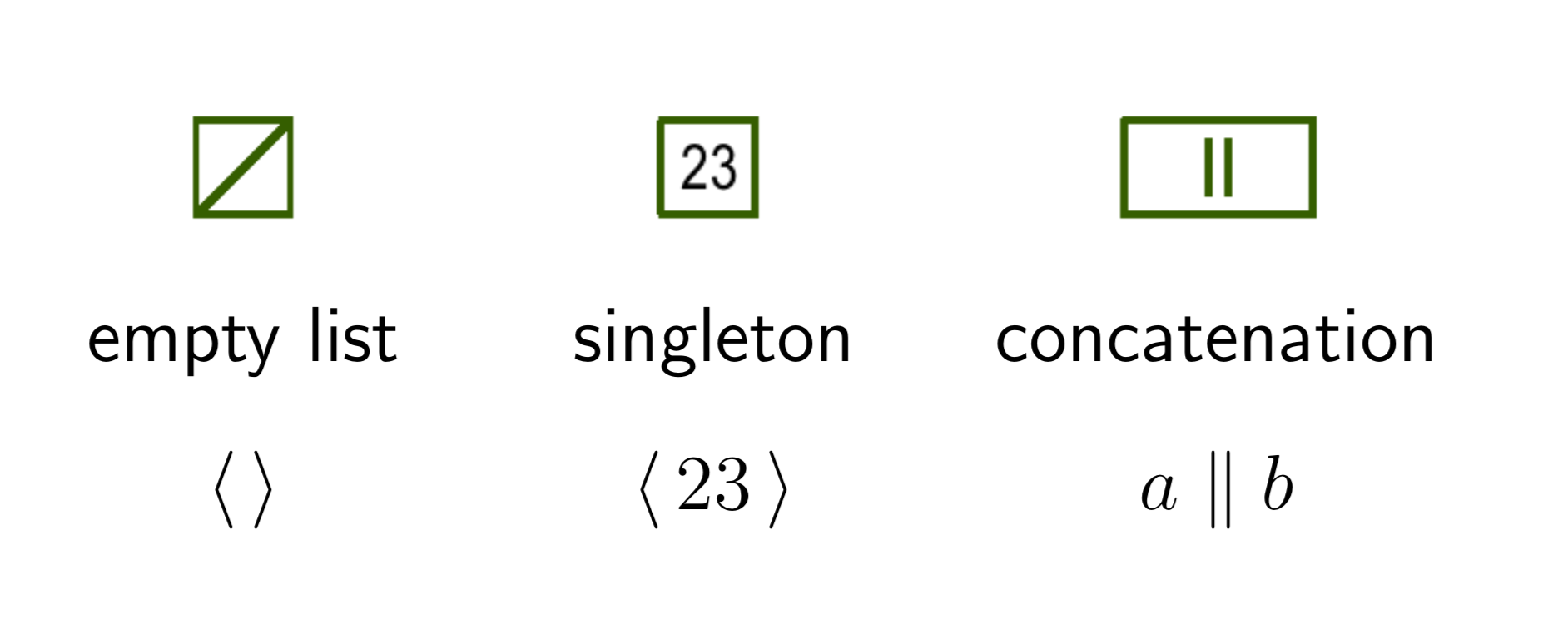Introduced by Guy Steele in Fortress

Conc List implementations available in

• Fortress
• Scala

This talk is about a `conc-list` implementation in Haskell

Simple inductive structure

``````data List a =
Empty
| Singleton a
| Conc (List a) (List a)``````
``````length :: List a -> Int
length Empty = 0
length (Singleton a) = 1
length (Conc xs ys)
= length xs + length ys -- potentially parallelizable``````

What happens when you filter?

``````filter :: (a -> Bool) -> List a -> List a
filter _ Empty = Empty
filter p (Singleton x)
| p x       -> (Singleton x)
| otherwise -> Empty
filter p (Conc xs ys) = Conc (p xs) (p ys)``````
``````let l =
Conc
(Conc (Singleton 23)
(Singleton 47))
(Conc (Singleton 18)
(Singleton 11))

filter (x > 15) l
=> Conc
(Conc (Singleton 23)
(Singleton 47))
(Conc (Singleton 18)
Empty)``````

`Conc lists` allow multiple possible represrentations of the same list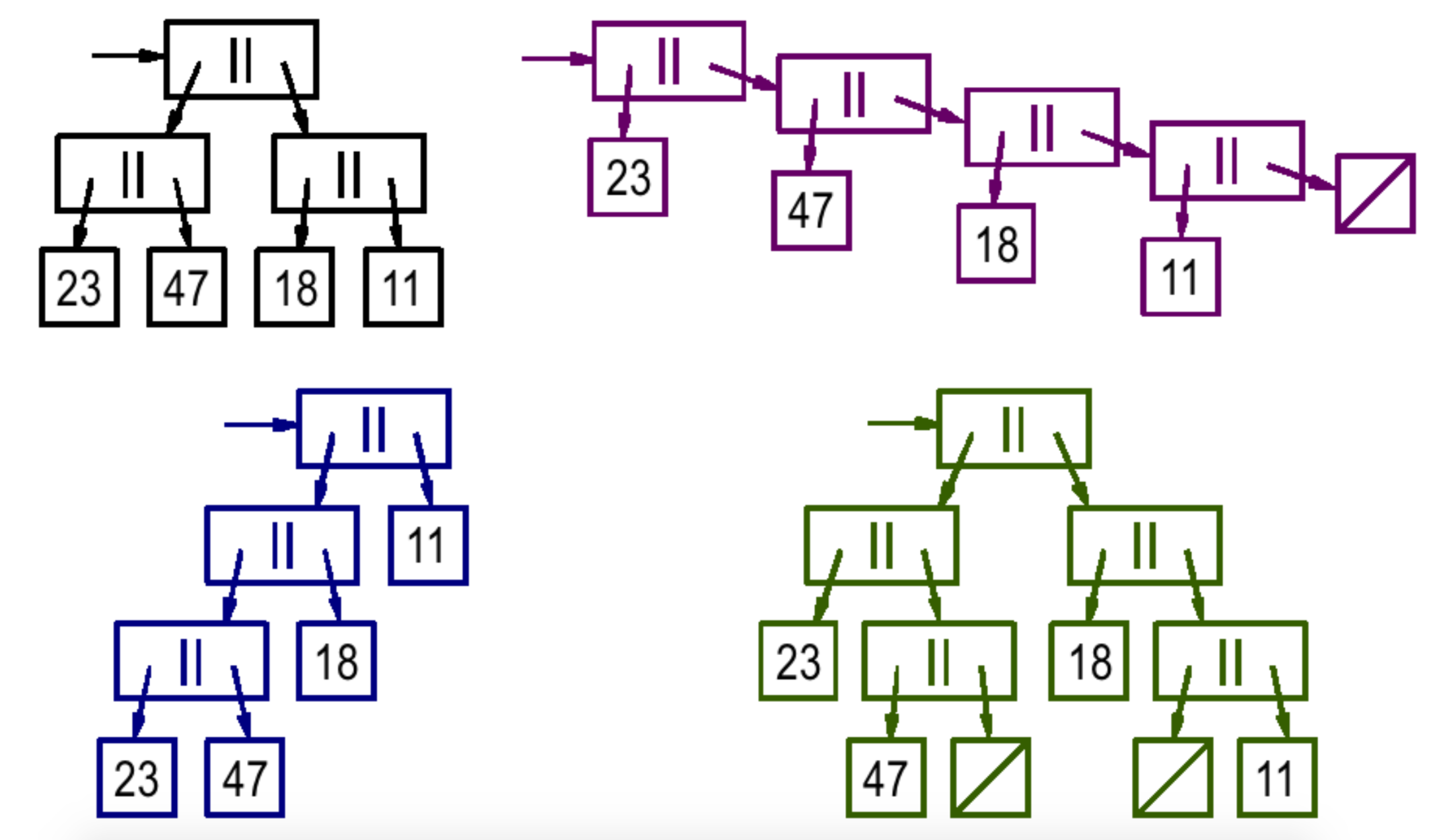Operations like filter spoil the "divide and conquer" like quality of the structure

We need a balanced tree.

``````type List a = Tree a

data Color = R | B deriving Show

data Tree a
= E
| S a
| C Color (Tree a) (Tree a)``````

`insert` on an RB Tree is quite simple.

`delete` is quite complex.

The core API: `cons`, `car`, `cdr`

``````cons :: Ord a => a -> List a -> List a
cons = insert

head :: List a -> Maybe a
head (S x) = Just x

tail :: Ord a => List a -> Maybe (List a)
tail E = Nothing
tail (S x) = Nothing
tail (C _ l r)
= let left_tail = fromMaybe E (tail l)
in Just \$ conc left_tail r``````

`conc` is slightly tricky

``````conc :: Ord a => List a -> List a -> List a
conc E E     = E
conc E (S a) = S a
conc E (C c l r) = C c l r
conc (S a) E = S a
conc (S a) (S b) = insert a (S b)
conc (S a) (C c l r) = insert a (C c l r)
conc (C c l r) E = C c l r
conc (C c l r) (S a) = balance B (C c l r) (S a)
conc (C c1 l1 r1) (C c2 l2 r2) = balance B (C c1 l1 r1) (C c2 l2 r2)
-- final implementation makes conc O(log n)``````

And also,

``````type List a = Tree a

instance Ord a => Semigroup (List a) where
(<>) = conc

instance Ord a => Monoid (List a) where
mempty = E
mappend = (<>)``````

`conc (left xs) (right xs) = xs`

Instead of implementing every function from Prelude in a specific way lets implement a general function.

``````foldMap :: Monoid m
=> (a -> m)
-> List a -- the actual structure
-> m
foldMap _ E = mempty
foldMap f (S x) = f x
foldMap f (C _ ys zs) = mappend (foldMap f ys) (foldMap f zs)``````
``````
length = getSum . foldMap (\_ -> Sum 1)

map f xs = foldMap (\x -> S (f x)) xs

filter p = foldMap (\x -> if p x then (S x) else E)

fold = foldMap id``````

How do we parallelize the operations?

``````foldMap :: Monoid m
=> (a -> m)
-> List a -- the actual structure
-> m
foldMap _ E = mempty
foldMap f (S x) = f x
foldMap f (C _ ys zs) = par2 mappend (foldMap f ys) (foldMap f zs)

par2 :: (a -> b -> c) -> a -> b -> c
par2 f x y = x `par` y `par` f x y``````

`par` is the simplest way to write pure parallel operations

``````par :: a -> b -> b
par x y = case (par# x) of { _ -> lazy y }``````

What is the problem with this?

• `(<>) = conc` is too costly

We need to restrict the parallelism

### HEIGHT ANNOTATED RED BLACK TREE

``````data Tree a
= E
| S a
| C {-# UNPACK #-} !Height
!Color
!(Tree a)
!(Tree a)``````

Now we can restrict the parallelism

``````foldMap :: Monoid m
=> (a -> m)
-> List a -- the actual structure
-> m
foldMap f t@(C h' _ _ _) = go t (h' - (logBase 2 h'))
where
go E _ = mempty
go (S x) _ = f x
go (C h _ ys zs) parallelDepth
| h >= parallelDepth = gol `par` gor `par` mappend gol gor
| otherwise = mappend gol gor
where
gol = go ys (n + 1)
gor = go zs (n + 1)``````

A more sensible parallelDepth magnitude

Plot a graph between performance and depth of throttling the parallelism

Can suggest a correlation between the two

JITed runtimes play with this sort of numbers

If we dynamically accept the mappend function

``````foldMap' :: (a -> m)
-> (m -> m -> m)
-> m
-> List a -- the actual structure
-> m
foldMap' f g unit t@(C h' _ _ _) = go t (h' - (logBase 2 h'))
where
go E _ = unit
go (S x) _ = f x
go (C h _ !ys !zs) parallelDepth
| h >= parallelDepth = gol `par` gor `par` g gol gor
| otherwise = g gol gor
where
gol = go ys (n + 1)
gor = go zs (n + 1)``````

We can reverse in parallel

``````reverse :: Ord a => List a -> List a
reverse = foldMap' S (\ys zs -> conc zs ys) E``````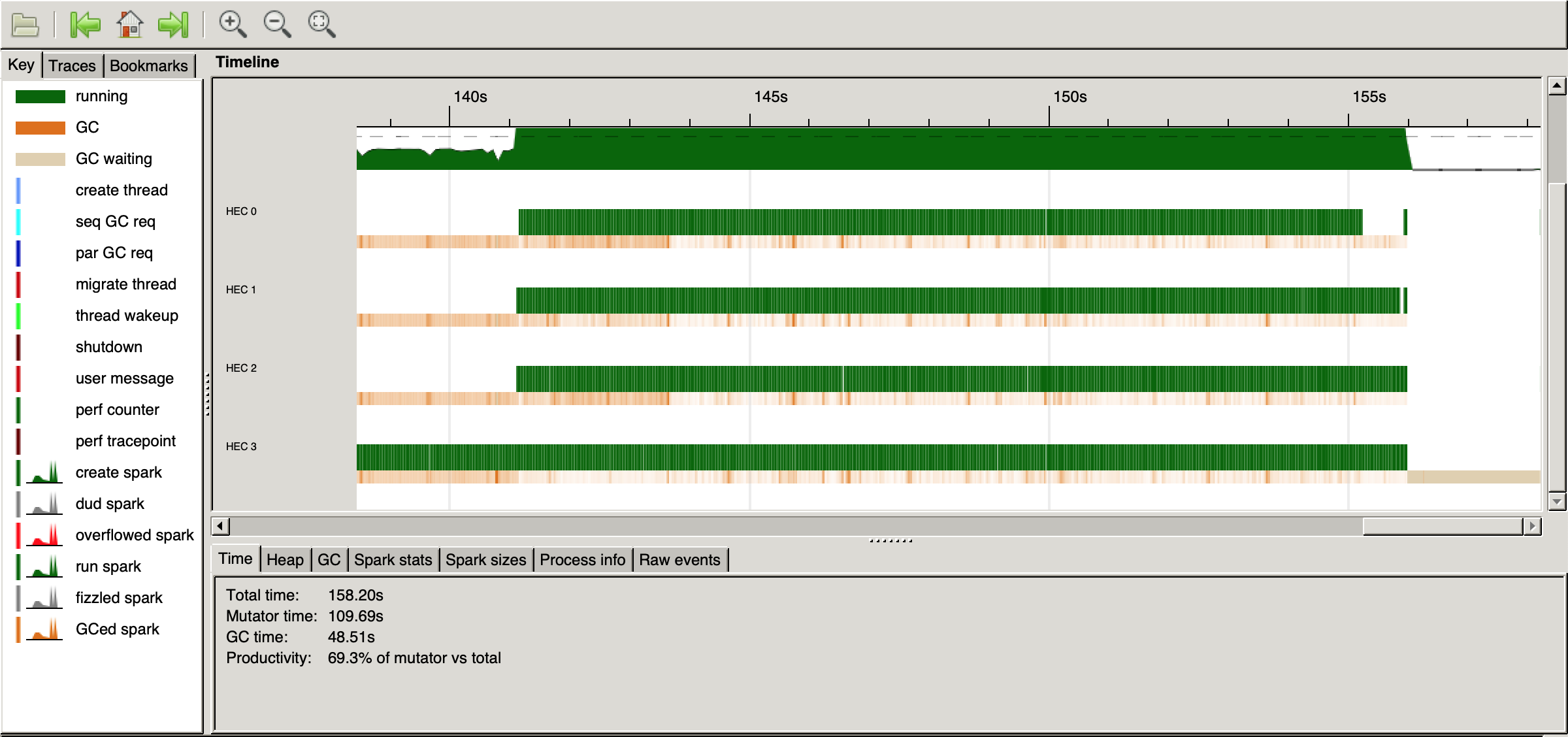### SHORTCOMINGS

• Space usage (for 10 million elements)

Height annotated RB Trees

``````  16,554,468,768 bytes allocated in the heap
2,715,002,064 bytes copied during GC
615,645,336 bytes maximum residency (12 sample(s))
1,494,888 bytes maximum slop
1204 MB total memory in use (0 MB lost due to fragmentation)``````

Lists

``````     720,127,264 bytes allocated in the heap
1,069,984 bytes copied during GC
95,992 bytes maximum residency (2 sample(s))
55,560 bytes maximum slop
5 MB total memory in use (0 MB lost due to fragmentation)``````
• List Fusion still more efficient
``````> length [0..10_000_000]

Rec {
lim_r3Bl = 10000000
lvl_r3Bm = 1

Foo.\$wgo :: Integer -> GHC.Prim.Int# -> GHC.Prim.Int#
Foo.\$wgo
= \ w_s3Ax ww_s3AB ->
case gtInteger# w_s3Ax lim_r3Bl
of {
__DEFAULT ->
Foo.\$wgo
(plusInteger w_s3Ax lvl_r3Bm) (+# ww_s3AB 1#);
1# -> ww_s3AB
}
end Rec }``````

### POSSIBLE IMPROVEMENTS

• Increase the branching factor

Eg: Clojure's persistent lists have a branching factor of 32

`````` -- A conc-rose tree
data NTree a
= E
| S a
| C !Height [NTree a]``````

### CONCLUSION

“To make the program faster, we have to gain more from parallelism than we lose due to the overhead of adding it, and compile-time analysis cannot make good judgments in this area.” - Simon Marlow